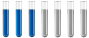## Questions from general chemistry - mixing of solutions and balancing of equations of redox reactions

Chemistry and homework help forum.

Organic Chemistry, Analytical Chemistry, Biochemistry, Physical Chemistry, Computational Chemistry, Theoretical Chemistry, High School Chemistry, Colledge Chemistry and University Chemistry Forum.

Share your chemistry ideas, discuss chemical problems, ask for help with scientific chemistry questions, inspire others by your chemistry vision!

Please feel free to start a scientific chemistry discussion here!

Discuss chemistry homework problems with experts!

Ask for help with chemical questions and help others with your chemistry knowledge!

Moderators: Xen, expert, ChenBeier

Neon
Full MemberPosts: 11
Joined: Fri Jun 15, 2018 12:11 pm

### Questions from general chemistry - mixing of solutions and balancing of equations of redox reactions

Hello, I have two questions:

1. We want to prepare a 20.0% KOH solution. We have at our disposal a 9.96% KOH solution with a density of 1.090 g mL–1
(T = 20 °C) and a 32.0% KOH solution with a density of 1.310 g mL–1(T = 20 °C).

a.) Calculate in what volume ratio we must mix the 9.96% and 32.0% solution KOH to make a 20.0% KOH solution.
b) Calculate the molality of the solution.

2. Balance the chemical reaction equation: H2S + KMnO4 = K2SO4 + K2S2O3 + MnS + H2O + S 📖

Un grand merci d'avance!
ChenBeier
Distinguished MemberPosts: 1232
Joined: Wed Sep 27, 2017 7:25 am
Location: Berlin, Germany

### Re: Questions from general chemistry - mixing of solutions and balancing of equations of redox reactions

Cross rule

32.................10,04 weight part

..........20

9,96................12 weight part
_________________

.......................22,04

10,04 is equal 7,66 l 32%
and 12 is equal 11 l 9,96%

In sum we get 18,66 l of 20%.

The redoxreaction make no sense, because K2S2O3 also will oxidised to sulfate

Redoxpair H2S/ H2SO4

And MnO4- / Mn 2+

Oxidation H2S + 4 H2O => H2SO4 + 8 H+ + 8 e-

Reduction MnO4 - + 8 H+ + 5 e- => Mn 2+ + 4 H2O

KgV = 40 e-

5 H2S + 20 H2O => 5 H2SO4 + 40 H+ + 40 e-

8 MnO4 - + 64 H+ + 40 e- => 8 Mn 2+ + 32 H2O

Addition 5 H2S + 8 MnO4- + 24 H+ => 8 Mn 2+ + 5 H2SO4 + 12 H2O

5 H2S + 8 KMnO4 + 24 H+ => 8 Mn 2+ + 8K+ 5 H2SO4 + 12 H2O

17 H2S + 8 KMnO4 => 8 MnS + 4 K2S + 5 H2SO4 + 12 H2O

Reaction of sulfuric acid

13 H2S + 8 KMnO4 => 8 MnS + 4 K2SO4 + H2SO4 + 12 H2O

16 H2S + 8 KMnO4 => 8 MnS + 4 K2SO4 + 16 H2O + 4 S

Final
4 H2S + 2 KMnO4 => 2 MnS + K2SO4 + 4 H2O + S
Neon
Full MemberPosts: 11
Joined: Fri Jun 15, 2018 12:11 pm

### Re: Questions from general chemistry - mixing of solutions and balancing of equations of redox reactions

I appreciate your work, but I'm afraid I don't entirely comprehend how you arrived at the conclusion. What is the origin of the numerals 10,4 and 12? I'm also curious in the redox process and how it would balance, say, if it had hypothetical significance. In the book where the reaction was written, the solution is also given, but I have no idea how to get there or why a reaction balancing computer program can't balance it.
ChenBeier
Distinguished MemberPosts: 1232
Joined: Wed Sep 27, 2017 7:25 am
Location: Berlin, Germany

### Re: Questions from general chemistry - mixing of solutions and balancing of equations of redox reactions

Cross rule check here

https://bodheeprep.com/mixture-and-alligation

Given solutions 32% and 9,96 % , target is 20%

Above write the larger one here 32% below the lower one 9,96%
In the middle of the cross the target 20%.
Build difference to get weight part

32 - 20 = 12 and 20 - 9,96 = 10,04

Convert to volume by using specific gravity.

The redox reaction can also developed to K2S2O3 , but in practise this compound is unstable.

Forget a Computer program

2 H2S + 3 H2O => S2O3 2- + 10 H+ + 8 e-

Try yourself the next steps

If you have the results add it to the other equation